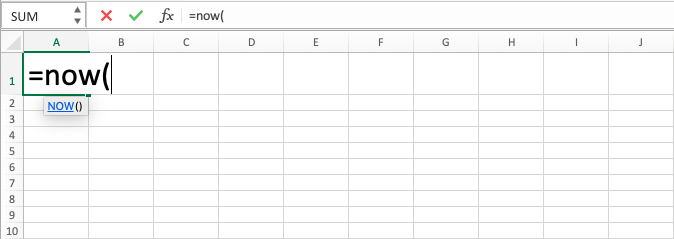Excel NOW Function - Compute Expert

# Excel NOW Function

In this tutorial, you will learn about NOW formula in excel. Excel NOW function can help us getting current date and time data to be placed in a cell or used in the calculation process.

## Why We Need to Learn About NOW Formula in Excel?

In spreadsheet software, sometimes we need detail recording on current time so it can be a note in the document and also probably as a processing factor in determining how long something has been operating if we have the data of when the operation started. That data is needed to do updated tracking continuously on something that does need that tracking process until the minute detail.

As a case example, imagine if we want to send a document that needs approval and the content is taken from a spreadsheet. Probably, we need to know the detail of when the document is created from the spreadsheet and sent, on what hour and what minute, as a documentation and also as an information note that will be taken a look at by the stakeholders who get the document so it can be a time benchmark on when should the feedback for this document needs to be sent.

As another example, currently, we probably monitor the production status of factory machines in a spreadsheet. There is a time when we need to monitor how long each machine has been operating so it can be determined faster when each of them needs to be rested so it will not have a burnout. Probably we can monitor them more accurately also on how many production results they can do in the period until the current time by having the current date, hour, and minute data which will be updated continuously on our spreadsheet.

To help in getting data on the most updated current date, time, and hour, then you can use excel NOW function. NOW formula in excel is very useful when facing cases like above where there is a need for current time data which is needed to be updated periodically.

## What is Excel NOW Function?

Excel NOW function is a function with the usage objective to give the data of current date, hour, and minute that keeps being updated. The update process of NOW formula in excel is done when you enter or update the content of the sheet where excel NOW function is.

## How to Use NOW Formula in Excel?

The following will explain how to write excel NOW function. NOW formula in excel is very easy to use because, like TODAY, it does not need input and you only need to write it directly where there is a need for current time data.

## Using Excel NOW Function

1. Type equal sign ( = ) in the cell where you want to put the result in2. Type NOW (can be with large and small letters) and open bracket sign after =3. Type close bracket sign4. Press Enter
5. The process is done!## Exercise

After you have learned how to use NOW formula in excel above, you can practice your understanding by doing the exercise below!

### Instruction

Answer all the questions in column B by using NOW formula in excel! Place your answers on column C

• You can use F9 button too to update NOW formula in excel result
• If there is a need to do a calculation of hour and minute with the result of this formula, then you need to write the hour and minute data and excel NOW function on two separate cells before they are combined. The process cannot be done directly in one writing
• If there is a need for today’s date which does not keep being updated continuously, then you can press Ctrl + ; at the same time to get the date ( Control and ; buttons on Mac)
• If there is a need for current time which does not keep being updated continuously, then you can press Ctrl + Shift + : at the same time to get the time ( Control, Shift, and : buttons on Mac)
• You can change the date and time format by using Format Cells menu or TEXT formula. If you want to use TEXT function, then you can learn it from the other part of Compute Expert blog if you still don’t understand how to use it!
• If there is a need for today’s date not including the time, you can use TODAY formula. The tutorial is also available in this Compute Expert blog!

Get updated excel info from Compute Expert by registering your email. It's free!